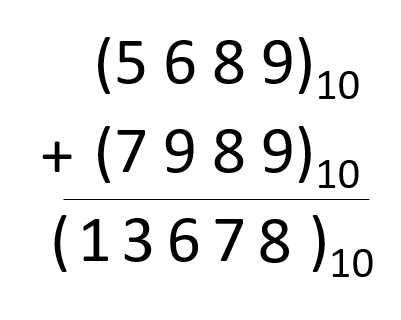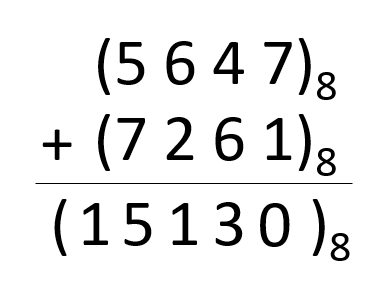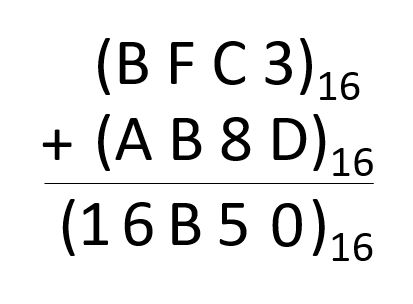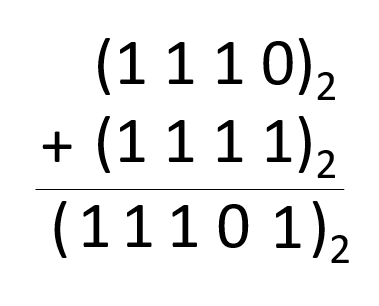# Third Chapter Lesson-5: Addition & Subtraction of numbers in different number systems.

## At the end of this lesson-

• 1. You will be able to do addition in different number systems.
• 2. You will be able to do Subtraction in different number systems.

## Go for Bangla Version

### Addition of different number system:

1. If summation of decimal digits is equal or more than the base of decimal number system, subtract 10(base) from summation( continue subtraction until summation comes to less than 10 )

2. Carry is how many times subtraction is occurred

Example: Addition of (5689)10 and (7989)101. If summation of octal digits is equal or more than the base of octal number system, subtract 8(base) from summation( continue subtraction until summation comes to less than 8)

2. Carry is how many times subtraction is occurred

Example: Addition of (5647)8 and (7261)81. If summation of hexadecimal digits is equal or more than the base of hexadecimal number system, subtract 16(base) from summation( continue subtraction until summation comes to less than 16)

2. Carry is how many times subtraction is occurred

Example: Addition of (BFC3)16 and (AB8D)161. If summation of binary digits is equal or more than the base of binary number system, subtract 2(base) from summation( continue subtraction until summation comes to less than 2)

2. Carry is how many times subtraction is occurred

Example: Addition of (1110)2 and (1111)2## Lesson Evaluation-

Knowledge Based Questions:

Comprehension Based Questions:

Creative Questions:

According to the stem answer the questions:

The ICT teacher came to class and wrote two numbers (72)8 and (3D)16 on the board. He then converted the numbers into two different number systems.

c) Express the sum in decimal number by adding the numbers given in the stem.

According to the stem answer the questions:

Tom, Jerry and Dora went to Newmarket to shop for the Eid, and they bought clothes by (10110)2, (76)8 and (53)16 taka respectively.

c) Determine the total price of Jerry and Dora’s clothes in  hexadecimal number system.

Multiple Choice Questions:

1. What is the subsequent number of 177 in octal number?

a) 100    b) 200      c) 170      d) 270

2. Which number will be in ? of the following hexadecimal series?

8, 9, A, B, C, D, E, F, ?

a) 01   b) 16   c) 0F    d) 10

3. What is the previous value of (10)16 ?

a) 9    b) A     c) E    d) F

4. What is the subsequent value of the series 1,8,F ?

a) A      b) B       c) 16      d) 22

5. What is the subsequent number of EFF ?

a)100      b) 200       c) F00      d) FF0

6. The value of (A)16+(10)2+(7)can be–

i. (13)16 ii. (23)iii. (10011)2

Which one is correct?

a) i & ii        b) i & iii     c) ii & iii       d) i, ii & iii

7. (100)2 + (AA)16 = ?

a) 1AA         b) 1B       c) AF     d) AE

8. Which one is equivalent value of (A+B+C)16 ?

a) (33)10  b) (100001)c) (ABC)16     d) (CBA)16

9. (1011.11)2 + (1101.10)2 ?

a) 10111.10      b) 11010.10     c) 11111.11  d) 11001.01

Written by,